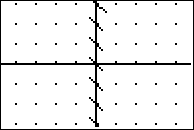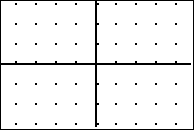••• ##### Device
• TI-83 Plus Family
• TI-84 Plus
• TI-84 Plus Silver Edition
•TI-84 Plus C Silver Edition
•TI-84 Plus CE
• ##### Report an Issue

Calculus: Introduction to Slope Fields
by Texas Instruments#### Overview

In this activity, students learn what a slope field represents in terms of dy/dx. Students create a slope field for a given differential equation

#### Key Steps

•Students will understand what a slope field represents in terms of the differential equation.

•Students will create a slope field for a given differential equation.